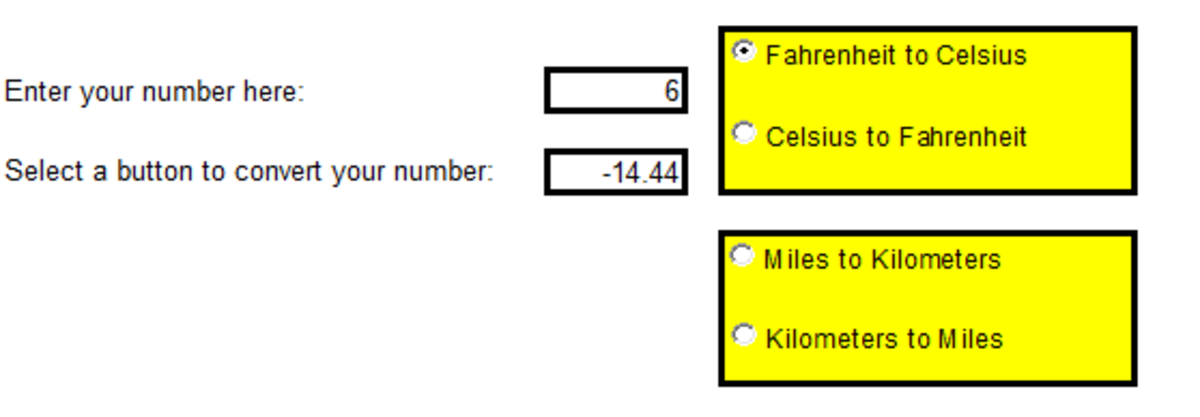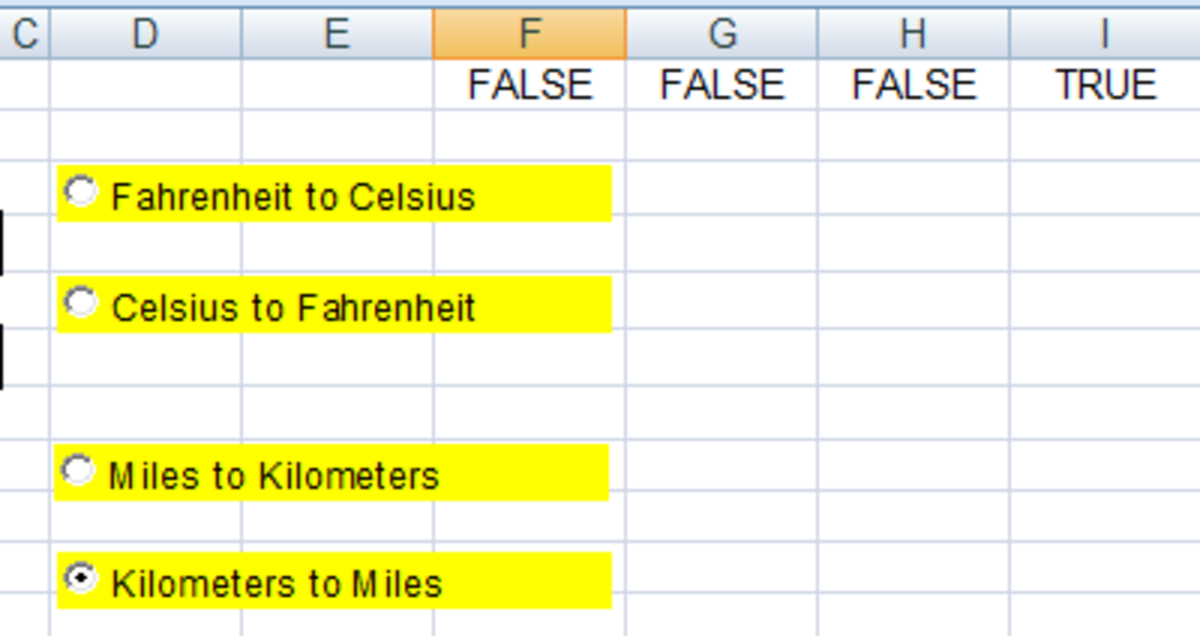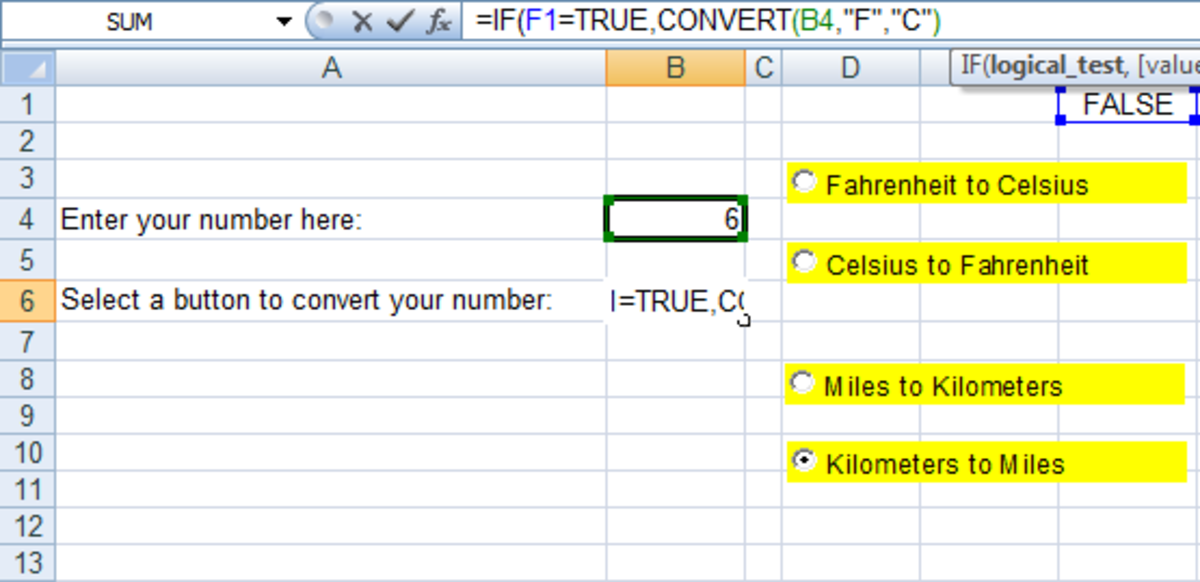# Using the CONVERT Function in Formulas in Excel 2007 and 2010

Robbie mostly writes about Skyrim but also occasionally sheds light on the oddities of Microsoft applications such as Excel and Outlook.

In this article, I am going to look at how to use the CONVERT function in formulas. The CONVERT function, as its name implies, is used to convert numbers from one measurement to another. So, you could convert a number from Celsius to Fahrenheit or from pounds to kilos for example.

You can convert a number from a large number of measurements as shown in the table below.

## Measurements That Can Be Used With the CONVERT Function and Some Examples

MeasurementConversions Available Include

Weight and Mass

Grams, Pounds and Ounces

Distance

Meters, Miles, Inches and Feet

Time

Minutes, Hours and Seconds

Pressure

Pascals and Atmospheres

Force

Newtons and Pound force

Energy

Joules and Calories

Power

Horsepower and Watts

Magnetism

Celsius and Fahrenheit

Liquid Measure

Teaspoons and Gallons

You can also add prefixes to metric units such as tera, giga or peta. So you could convert Gigawatts to Horsepower, or Kilojoules to a Thermodynamic calorie using the CONVERT function.

In today’s article, we will work through two examples.

• The first is to use the convert function in a formula to convert Fahrenheit to Celsius and also Kilometres to Miles.
• The second is to use the CONVERT function with Option (or Radio) Buttons and IF functions to create a panel where someone can enter a number and choose to convert it into a number of different measurements as shown below.Using the CONVERT function with Option (Radio) buttons to create a panel which allows users to convert numbers between various measurements automatically.http://robbiecwilson.hubpages.com/

## Using the CONVERT Function in a Formula

The CONVERT function is fairly straightforward and quite easy to use. In our first example, we will convert Celsius to Fahrenheit. The CONVERT function has three variables:

• The cell that contains the number you want to CONVERT (in my example, B4).
• What you want to CONVERT it from (in this case, Celsius).
• What you want to CONVERT it to (in my example, Fahrenheit).

The complete formula for my example is:

=CONVERT(B4,"C","F")

For the above formula, C=Celsius and F=Fahrenheit.

In my next example, we will convert kilometres to miles. In this case, the formula will be:

=CONVERT(B4,"km","mi")

In this case, k=kilo and m=metre and mi=miles.

Scroll to Continue

Note: the k is a prefix which means 1,000, so km is 1,000 metres rather than 1 km.

To perform a conversion using different measurements, simply change what you want to change it from and what you want to change it to.

## Using Option/Radio Buttons With IF Functions and the CONVERT Function to Allow Users to Choose a Conversion

In the next example, we are going to combine the CONVERT function with Option or Radio buttons and the IF function to create an interactive panel where users can select the conversion they want to use and Excel will automatically convert a number of their choosing. Ultimately, we will end up with a panel looking something like mine above.

To start, we will create the Option (or Radio) buttons. First, you need to have the Developer tab visible. In Excel 2007, to enable it should it be missing:

• Navigate to the Excel button.
• Click Excel Options.
• Choose the Popular tab, select Show Develop tab in the Ribbon.

For Excel 2010, adding the Developer tab is different.

• Click on the File menu.
• Select Options.
• Choose the Customise Ribbon tab.
• Select Developer in the Main Tabs section (illustrated below with the red arrow).

To create an Option Box, click on the Developer tab then navigate to the Insert button in the Controls group and select Option Button in the ActiveX Controls section.

• The cursor will change to a cross. Draw the outline of your button.
• Now we have to copy it to create the number of buttons you need. To do this, select your Option button, right click choose Copy and then Paste.
• Next we need to configure the button, select your button and then choose the Properties button in the Controls group.

You will want to configure several of these options.

• BackColor: this changes the colour of the button itself (I made mine yellow).
• Caption: is the text that appears on your button.
• Height and Width: allows you to ensure your buttons are all identical in size.
• LinkedCell: this is the cell that Excel will use to report whether your button has been selected (True) or not (False).

The other options can be left as is (to learn more about these options, my article mentioned above goes into these options in far more detail).

Note: each of your Option (Radio) buttons must have a different linked cell, or they will not work properly.

You can see from the below figure, I have set up four Option buttons with their linked cells F1:I1. I1 is set to TRUE as the button it is linked to has been selected.The Option (or Radio) buttons for the measurement conversion panel have been created and configured using Excel 2007 and Excel 2010.http://robbiecwilson.hubpages.com/

## Converting the Option (Radio) Buttons Output Using Nested IF Function in a Formula

The next step is to take the output from our buttons and using IF, convert the user’s number using the measurements they selected.

• IF will look at each of the linked cells to see if they are true (in other words selected by the user of the spreadsheet).
• If they are, Excel will use a CONVERT function to transform the user’s number using the conversion they selected.

The IF function is a very powerful and versatile function. I have a number of articles on the IF function, including:

• SUMIF is used to SUM numbers IF they meet a single criteria (for example, sum all sales if they were made by Jim) and SUMIFS which is used when you have more than one criterion (for example, sum all sales made by Jim in October).
• COUNTIF and COUNTIFS work in a similar manner, except it performs a count rather than a sum of the data. COUNTIF for example, could be used to count the number of times Sammy has been on detention and COUNTIFS could count the number of detentions he had in November.
• Lastly, I have an article that looks at the IF and IFERROR functions in great detail, investigating how to nest IF functions and also how to us it with AND, OR and NOT so that you can complex create formulas (for example IF a cell contains Sales AND another contains Jim then SUM a specific cell). IFERROR allows you to suppress known errors. This article can be found here.

The formula we will produce is quite long, so we will break it down into bite sized chunks to better understand how it works. The first part (stored in cell B6) is:

=IF(F1=TRUE,CONVERT(B4,"F","C")

• F1 is the linked cell for our first Option button, so if the user selects it, then F1 will be TRUE, otherwise it will be FALSE
• B4 is the cell that the number being converted is entered into.
• “F” stands for Fahrenheit and “C” for Celsius.

So, if the user chooses to CONVERT Fahrenheit to Celsius by selecting its Option button then Excel will convert B4 from Fahrenheit to Celsius

You can see this illustrated below (I have removed the rest of the formula to make it less confusing).Example of the first part of nested IF functions performing a conversion in Excel 2007 and Excel 2010.http://robbiecwilson.hubpages.com/

Using nested IF functions, I add the formulas for the other three Option (or Radio) buttons to the formula:

,IF(G1=TRUE,CONVERT(B4,"C","F") – Converts B4 from Celsius to Fahrenheit

,IF(H1=TRUE,CONVERT(B4,"mi","km") – Converts B4 from miles to kilometres

,IF(I1=TRUE,CONVERT(B4,"km","mi"))))) – Converts B4 from kilometres to miles

So the whole formula when joined together looks like this:

=IF(F1=TRUE,CONVERT(B4,"F","C"),IF(G1=TRUE,CONVERT(B4,"C","F"),IF(H1=TRUE,CONVERT(B4,"mi","km"),IF(I1=TRUE,CONVERT(B4,"km","mi")))))

Finally, I used borders and filled the cells yellow to complete my panels. To do this:

• Select the cells you wish to format as a block.
• Right click and select Format Cells (ensure that you are selecting the cells and not the Option (Radio) buttons, your cursor will be a white cross if you are selecting the cells and a smaller black cross if you are selecting the buttons).
• On the Border tab, select the border you want to use and select Outline.
• Next, click on the Fill tab and choose a colour to compliment the colour of your Option buttons.
• Click OK to close the dialogue box.

To ensure that your linked cells are not accidentally changed or deleted, I would also recommend that you hide the row that they are on (in my case Row 1) by selecting the row and right clicking and selecting Hide.

## Conclusion

Today, we have looked at the very useful CONVERT function. It allows you to CONVERT the contents of a cell into any number of different measurements. It can convert weights, distances, time and temperature as well as a number of other measurement types.

We then looked at a couple of examples of converting a number from Fahrenheit to Celsius and also Kilometres to Miles.

Then, we did something that I really enjoy doing and something that Excel really does well. We took the CONVERT function, the IF function and Option (or Radio) buttons and combined them together to create a panel that allows the users of your spreadsheet to enter a value and choose which measurements they wanted it converted into and have the spreadsheet do it all for them automatically and seamlessly.

I do hope that you have found today’s article useful and informative and that you know more about how and when to use the CONVERT function in Excel 2007 and 2010. Many thanks for reading and feel free to leave any comments you may have in the box below.